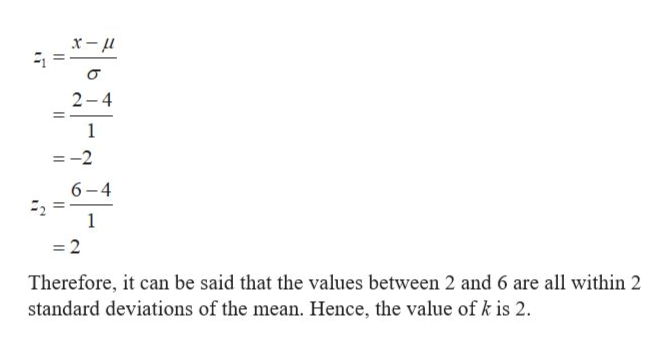# From a sample with n=32, the mean number of televisions per household is 4 with a standard deviation of 1 television. Using Chebychev's theorem, determine at least how many of the households have between 2 and 6 televisions.

Question

From a sample with n=32, the mean number of televisions per household is 4 with a standard deviation of 1 television. Using Chebychev's theorem, determine at least how many of the households have between 2 and 6 televisions.

check_circle

Step 1

Chebyshev’s rule can be used for the large set data. It says that at least 1-1/k2 percentage of the data falls within the k standard deviation of the mean, where k is a positive number greater than 1. It can be represented as:

Step 2

According to the provided data, for a sample size, n is 32, the mean, µ is 4 with a standard deviation, σ of 1.

The number of standard deviations under which the values 2 and 6 are falling can be obtained by calculating the z-score.help_outlineImage Transcriptionclose2-4 1 =-2 6-4 =2 Therefore, it can be said that the values between 2 and 6 are all within 2 standard deviations of the mean. Hence, the value of k is 2 fullscreen
Step 3

Using Chebyshev’s Inequality, the percenta...

### Want to see the full answer?

See Solution

#### Want to see this answer and more?

Solutions are written by subject experts who are available 24/7. Questions are typically answered within 1 hour.*

See Solution
*Response times may vary by subject and question.
Tagged in

### Statistics